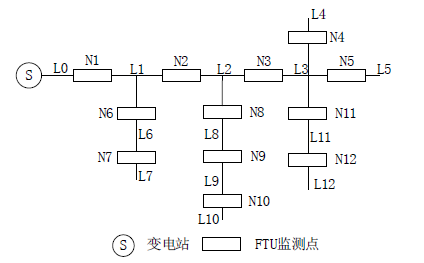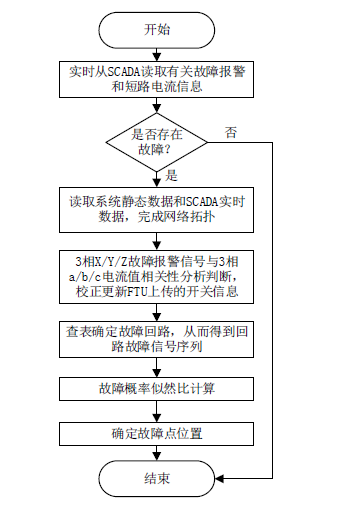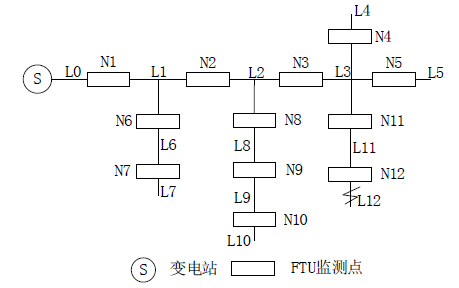﻿ 基于贝叶斯似然比形式的配电网故障定位新方法

# 基于贝叶斯似然比形式的配电网故障定位新方法A New Fault Location Method for Distribution Network Based on Bayes Likelihood Ratio

Abstract: In order to realize the fast fault-tolerant fault location of the distribution network under nonperfect fault information, a three-phase current information is put forward in the terminal opening space, and the main station is uploaded. After failure, reported by distribution network fault after the feeder switch remote communication between each phase fault alarm signal, remote measure be-tween three phase fault current and three-phase fault current with the phase correlation between fault alarm signal, omission, false alarm for the presence of terminal equipment to report the fault alarm signal correction. A fault addressing model based on this algorithm is established, and the Bayes likelihood ratio operation is performed to locate the fault in the maximum probability of failure. The algorithm is simple and practical, which can block the errors of SCADA data to a certain extent, and verify the accuracy and validity of the algorithm by an example.

1. 引言

2. 配电网区段定位算实现

2.1. 贝叶斯似然比定义

$P\left(B|A\right)=\frac{P\left(A|B\right)}{P\left(A\right)}P\left(B\right)$ (1)

$\frac{P\left({B}_{1}|A\right)}{P\left({B}_{2}|A\right)}=\frac{P\left(A|{B}_{1}\right)P\left({B}_{1}\right)}{P\left(A|{B}_{2}\right)P\left({B}_{2}\right)}$ (2)

2.2. 电网畸变故障报警信号的校正

$\left\{\begin{array}{l}{g}_{X}=1,{I}_{X}>{I}_{Z}\\ {g}_{X}=0,{I}_{X}<{I}_{Z}\end{array}$

1) 配电网馈线发生故障后，主站同时收集各馈线开关a、b、c三相的故障报警信号和遥测上传的三相电流信息。

2) 若主站定义的某一开关的X相的故障报警信号fX = 1，Y相的故障报警信号fY = 1，即该开关至少有两相故障报警信号为1，则判断该开关故障报警信号未发生畸变，其故障信息标志为f = 1，转至步骤5)；若主站定义的某一开关的三相故障报警信号均为0，则判断该开关故障报警信号未发生畸变，故障信息标志为f = 0，转至步骤5)；若主站定义某一开关的X相故障报警信号fX = 1，Y相和Z相的故障报警信号fY = 0、fZ = 0，即仅有一相的故障报警信号为1，则判断出该开关的故障报警信号发生畸变，需要对该开关畸变的报警信号进行误报或者漏报检验。

3) 将主站收集的该开关a、b、c三相的电流信息与整定值比较，同样定义，若该开关任一相电流大于系统电流整定值，则定义gX = 1；反之，则定义gX = 0：

a) 若存在故障报警信息畸变的开关fX = gX = gY = 1，fY = 0，则判断该开关Y相故障报警信号为漏报；

b) 若存在故障报警信息畸变的开关fY = gX = gY = 0，fX = 1，则判断该开关X相故障报警信号为误报。

4) 根据故障报警信号误报、漏报的检验结果，对存在误报或者漏报的故障报警信号进行修正，给出健全的故障信息。

a) 若该开关Y相存在故障报警信号漏报，则将该开关Y相故障报警信号修正为1，即fY = 1，最后得到该开关故障信息标志为f = 1；

b) 若该开关X相存在故障报警信息误报，将该开关X相故障报警信号修改为0，即fX = 0，最后得到该开关故障信息标志为f = 0。

5) 根据修正后的各开关的故障信息标志f，最终得到故障信号序列 $F=\left({f}_{1},{f}_{2},\cdots ,{f}_{n}\right)$

2.3. 配电网的故障区段定位

2.3.1. 配电网络拓扑结构定义

1) 支路末端：除独立电源外，只和一个开关关联的支路如图1中的 ${L}_{4}$

2) 回路：从电源节点沿馈线向线路末端供电所经历的馈线开关的有序集合。如 $\left[{L}_{0},{L}_{1},{L}_{2},{L}_{3},{L}_{5}\right]$ 构成一个回路。

3) 回路故障信号序列：SCADA系统接收到在回路各馈线开关上安装的检测装置上测得的故障信息，根据上传的故障指示信号形成有序集合 ${F}_{回路}=\left({f}_{1},{f}_{2},\cdots ,{f}_{n}\right)$ ，其中fi为按照上小节步骤校正后的值，1表示有故障信号，0表示无故障信号。

2.3.2. 故障回路判断

2.3.3. 故障概率似然比判别

1) 在SCADA系统中，每个开关上FTU报警的错误和向上通信时出错的概率是均等的，定义为p且 $p\ll 1$

3) 配电网中任何一条支路发生故障的概率都相同。Figure 1. The network diagram of distribution systemTable 1. Distribution network stratification

SCADA系统接收到故障回路中负荷开关 ${N}_{1},{N}_{2},\cdots ,{N}_{n}$ 传来的故障信号序列 ${F}_{回路}=\left({f}_{1},{f}_{2},\cdots ,{f}_{n}\right)$ ，半段支路 ${L}_{i}$ 发生故障的概率用 $P\left({N}_{i}|F\right)$ ，根据条件概率的贝叶斯公式：

$P\left({N}_{i}|F\right)=\frac{P\left({N}_{i}F\right)}{P\left(F\right)}=\frac{P\left(F|{N}_{i}\right)P\left({N}_{i}\right)}{P\left(F\right)}$ (3)

${\delta }_{ij}=\frac{P\left({N}_{i}|F\right)}{P\left({N}_{j}|F\right)}=\frac{\frac{P\left(F|{N}_{i}\right)P\left({N}_{i}\right)}{P\left(F\right)}}{\frac{P\left(F|{N}_{j}\right)P\left({N}_{j}\right)}{P\left(F\right)}}=\frac{P\left(F|{N}_{i}\right)P\left({N}_{i}\right)}{P\left(F|{N}_{j}\right)P\left({N}_{j}\right)}$ (4)

${\delta }_{ij}=\frac{P\left(F|{N}_{i}\right)}{P\left(F|{N}_{j}\right)}$ (5)

$P\left(F|{N}_{i}\right)={p}^{\left(\underset{j=1}{\overset{i}{\sum }}\stackrel{¯}{{f}_{j}}+\underset{j=i+1}{\overset{n}{\sum }}{f}_{j}\right)}{\left(1-p\right)}^{\left(\underset{j=1}{\overset{i}{\sum }}{f}_{j}+\underset{j=i+1}{\overset{n}{\sum }}\stackrel{¯}{{f}_{j}}\right)}$ (6)

1) ${\delta }_{ij}>1$ ，支路 ${L}_{i}$${L}_{j}$ 发生故障的可能性大；

2) ${\delta }_{ij}=1$ ，支路 ${L}_{i}$${L}_{j}$ 发生故障的可能性相等；

3) ${\delta }_{ij}<1$ ，支路 ${L}_{i}$${L}_{j}$ 发生故障的可能性小。

$\begin{array}{c}P\left({N}_{i}|F\right)=\mathrm{max}\left\{P\left({N}_{1}|F\right),P\left({N}_{2}|F\right),\cdots ,P\left({N}_{n}|F\right)\right\}\\ =\mathrm{max}\left\{P\left(F|{N}_{1}\right),P\left(F|{N}_{2}\right),\cdots ,P\left(F|{N}_{n}\right)\right\}\end{array}$ (7)

2.4. 算法实现流程Figure 2. Flow chart of fault location algorithm in distribution networkFigure 3. Distribution network with lasting fault occur to L12

3. 算例分析

$\begin{array}{c}P\left({N}_{i}|F\right)=\mathrm{max}\left\{P\left({N}_{1}|F\right),P\left({N}_{2}|F\right),P\left({N}_{3}|F\right),P\left({N}_{11}|F\right),P\left({N}_{12}|F\right)\right\}\\ =\mathrm{max}\left\{P\left(F|{N}_{1}\right),P\left(F|{N}_{2}\right),P\left(F|{N}_{3}\right),P\left(F|{N}_{11}\right),P\left(F|{N}_{12}\right)\right\}\\ =\mathrm{max}\left\{{p}^{4}\left(1-p\right),{p}^{3}{\left(1-p\right)}^{2},{p}^{2}{\left(1-p\right)}^{3},p{\left(1-p\right)}^{4},{\left(1-p\right)}^{5}\right\}\end{array}$ (8)

${\delta }_{ij}=\frac{P\left({N}_{12}|F\right)}{P\left({N}_{1}|F\right)}=\frac{P\left(F|{N}_{12}\right)}{P\left(F|{N}_{1}\right)}=\frac{{\left(1-p\right)}^{5}}{{p}^{4}\left(1-p\right)}=\frac{{\left(1-p\right)}^{4}}{{p}^{4}}$ (9)

$\begin{array}{c}P\left({N}_{i}|F\right)=\mathrm{max}\left\{P\left({N}_{1}|F\right),P\left({N}_{2}|F\right),P\left({N}_{3}|F\right),P\left({N}_{11}|F\right),P\left({N}_{12}|F\right)\right\}\\ =\mathrm{max}\left\{P\left(F|{N}_{1}\right),P\left(F|{N}_{2}\right),P\left(F|{N}_{3}\right),P\left(F|{N}_{11}\right),P\left(F|{N}_{12}\right)\right\}\\ =\mathrm{max}\left\{{p}^{3}{\left(1-p\right)}^{2},{p}^{2}{\left(1-p\right)}^{3},{p}^{3}{\left(1-p\right)}^{2},{p}^{2}{\left(1-p\right)}^{3},p{\left(1-p\right)}^{4}\right\}\end{array}$ (10)

${\delta }_{ij}=\frac{P\left({N}_{12}|F\right)}{P\left({N}_{1}|F\right)}=\frac{P\left(F|{N}_{12}\right)}{P\left(F|{N}_{1}\right)}=\frac{p{\left(1-p\right)}^{4}}{{p}^{3}{\left(1-p\right)}^{2}}=\frac{{\left(1-p\right)}^{2}}{{p}^{2}}$ (11)

4. 结论

 郭壮志, 徐其兴, 洪俊杰, 等. 配电网快速高容错性故障定位的线性整数规划方法[J]. 中国电机工程学报, 2016, 36: 1-9.

 牛耕, 周龙, 裴玮, 等. 基于克拉克电流相角差值的低压有源配电网故障定位方法[J]. 中国电机工程学报, 2015, 35(S): 15-24.

 张姝, 杨健维, 何正友, 等. 基于线路暂态重心频率的配电网故障区段定位[J]. 中国电机工程学报, 2015, 35(10): 2463-2470.

 倪广魁, 鲍海, 张利, 等. 基于零序电流突变量的配电网单相故障带电定位判据[J]. 中国电机工程学报, 2010, 30(31): 118-122.

 刘健, 赵倩, 程红丽, 等. 配电网非健全信息故障诊断及故障处理[J]. 电力系统自动化, 2010, 34(7): 50-56.

 刘健, 贠保记, 崔琪, 等. 一种快速自愈的分布智能馈线自动化系统[J]. 电力系统自动化, 2010, 34(10): 82-86.

 刘健, 赵树仁, 贠保记, 等. 分布智能型馈线自动化系统快速自愈技术及可靠性保障措施[J]. 电力系统自动化, 2011, 35(17): 67-71.

 焦振有, 焦邵华, 刘万顺. 配电网馈线系统保护原理及分析[J]. 电网技术, 2002, 26(12): 75-78.

 章琦. 基于面保护原理的配电网故障处理[J]. 浙江电力, 2002, 21(2): 14-17.

 刘文轩, 严凤, 田霖, 等. 基于LVQ 神经网络的配电网故障定位方法[J]. 电力系统保护与控制, 2012, 40(5): 90-95.

 刘健, 董新洲, 陈星莺, 等. 配电网容错故障处理关键技术研究[J]. 电网技术, 2012, 36(1): 253-257.

 郭壮志, 吴杰康. 配电网故障区间定位的仿电磁学算法[J]. 中国电机工程学报, 2010, 30(13): 34-40.

 王英英, 罗毅, 涂光瑜. 基于贝叶斯公式的似然比形式的配电网故障定位方法[J]. 电力系统自动化, 2005, 29(19): 54-57.

 郑涛, 潘美玉, 王英男, 等. 配电网具有容错性的快速故障定位方法研究[J]. 电力系统保护与控制, 2014, 42(6): 63-67.

Top